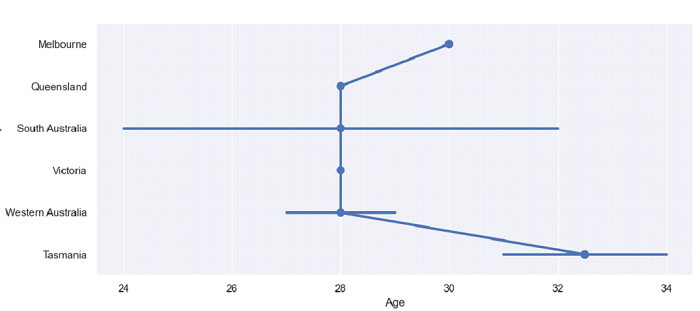# Python Pandas - Draw a set of Horizontal point plots with Seaborn

Horizontal point plots are a plotting based on the values of x and y i.e. the columns of the dataset you consider. Point Plot in Seaborn is used to show point estimates and confidence intervals using scatter plot glyphs. The seaborn.pointplot() is used for this.

Let’s say the following is our dataset in the form of a CSV file − Cricketers.csv

At first, import the required libraries −

import seaborn as sb
import pandas as pd
import matplotlib.pyplot as plt

Load data from a CSV file into a Pandas DataFrame −

dataFrame = pd.read_csv("C:\Users\amit_\Desktop\Cricketers.csv")

Now, use the pointplot() and set the x and y values −

sb.pointplot(x = 'Age', y = 'Academy', data = dataFrame)

## Example

Following is the code −

import seaborn as sb
import pandas as pd
import matplotlib.pyplot as plt

# Load data from a CSV file into a Pandas DataFrame

sb.set_theme(style="darkgrid")

# Horizontal point plot
sb.pointplot(x = 'Age', y = 'Academy', data = dataFrame)

# display
plt.show()

## Output

This will produce the following output −